Partial differential operators.

## Linear Methods of Applied Mathematics Evans M. Harrell II and James V. Herod*

version of 1 June 1996

## Partial differential operators - classification and adjoints.

Language and Classification

In this beginning with second order equations, we will take a look at the language of partial differential equations. We should be sure that we all have the same vocabulary. Since there is some standard language for the identification of partial differential equations, let's agree with the language that every one else uses as a start.

Several parts are easy. Suppose that we have a partial differential equation in u. The order of the equation is the order of the highest partial derivative of u, the number of variables is simply the number of independent variables for u in the equation, and the equation has constant coefficients if u and the coefficients of all the partial derivatives of u are constant. If all the terms involving u are moved to the left side of the equation, then the equation is called homogeneous if the right side is zero, and non homogeneous if it is not zero. The system is linear if that left side involves u in only a "linear way."

Examples:

(1) 4uxx - 24 uxy + 11uyy - 12ux - 9uy - 5u = 0

is a linear, homogeneous, second order partial differential equation in two variables with constant coefficients.

(2) ut = x uxx + y uyy + u2

is a nonlinear, second order partial differential equation in two variables and does not have constant coefficients.

(3) utt - uxx = sin( \pi t)

is a non-homogeneous equation.

In thinking of partial differential equations, it is a common practice to carry over the language that has been used for matrix or ordinary differential equations in as far as possible. Recall that one solved linear systems of algebraic equations such as the equation

3x + 4y = 0

5x + 6y = 0.

This equation could be re-written as a matrix system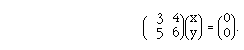One talked of the matrix equation or the linear homogeneous equation Au = 0, where u and 0 are understood to be vectors in the two dimensioned vector space on which the linear operator A is defined.

In a similar manner, in sophomore differential equations, one considered equations such as

x' = 3x + 4y

y' = 5x + 6y

and, perhaps, re-wrote these equations as a system z' = Az where A is the matrix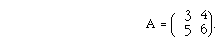This is a first order, linear system; A is a linear operator on R2 and we follow the vector value function u(t) as it runs through that space, changing in time as prescribed by the differential equation

du/dt = Az.

So, in partial differential equation, we consider linear equations

Lu = 0, or u' = Lu,

only now, L is a linear operator on a space of functions. For example, it may be that L(u) = uxx + uyy. And, a corresponding notion for the equation u' = Lu is ut = uxx + uyy.

The notion that a linear operator can have domain a space of functions may seem alien at first, but the analogies from Rn are more than superficial. This is an idea worthy of consideration.

We will be interested in a rather general second order, differential operator. In two variables, we consider the operator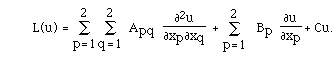(16.1)


There is an analogous formula for three variables.

We suppose that u is smooth enough so that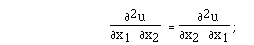(16.2)


that is, we can interchange the order of differentiation. In this first consideration, the matrix A, the vector B, and the number C do not depend on u; we take the matrix A to be symmetric. Because of (16.2) we are free to arrange this, since the coefficient of the cross term is A12 + A21, and we may assign A12 and A21 any way we wish, so long as the sum has the right value.

Example

We write an example in the matrix representation for constant coefficient equations:

L[u] = 4uxx - 24 uxy + 11uyy - 12ux - 9y - 5u

can be written as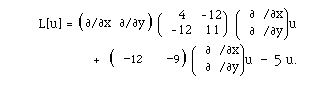We shall be interested in an equation which has the form

L(u) = f

or u' = L(u) + f.

In this section, u is a function on R2 or R3 and the equations are to hold in an open, connected region D of the plane. We will also assume that the boundary of the region is piece-wise smooth, and denote this boundary by \boundary D. Just as in ordinary differential equations, in partial differential equations some boundary conditions will be needed to solve the equations. We will take the boundary conditions to be linear and have the general form

B(u) = a u + b u\eta,

where u\eta is the derivative taken in the direction of a normal to the boundary of the region.

The techniques of studying partial differential operators and the properties of these operators change depending on the "type" of operator. These operators have been classified into three principal types. The classifications are made according to the nature of the coefficients in the equation which defines the operator. The operator is called an elliptic  operator if the eigenvalues of A are non-zero and have the same algebraic sign. The operator is hyperbolic  if the eigenvalues have opposite signs and is parabolic  if at least one of the eigenvalues is zero.

The classification makes a difference both in what we expect from the solutions of the equation and in how we go about solving them. Each type is modeled on an important equation of physics.

The basic example of a parabolic equation is the one dimensional heat equation. Here, u(t,x) represents the heat on a line at time t and position x. One should be given an initial distribution of temperature which is denoted u(0,x), and some boundary conditions which arise in the context of the problem. For example, it might be assumed that the ends are held at some fixed temperature for all time. In this case, boundary conditions for a line of length L would be u(t,0) = \alpha and u(t,L) = \beta . Or, one might assume that the ends are insulated. A mathematical statement of this is that the rate of flow of heat through the ends is zero: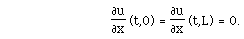The manner in which u changes in time is derived from the physical principle which states that the heat flux at any point is proportional to the temperature gradient at that point and leads to the equation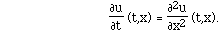Geometrically, one may think of the problem as one of defining the graph of u whose domain is the infinite strip bounded in the first quadrant by the parallel lines x = 0 and x = L The function u is known along the x axis between x = 0 and x = L, To define u on the infinite strip, move in the t direction according to the equation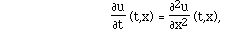while maintaining the boundary conditions.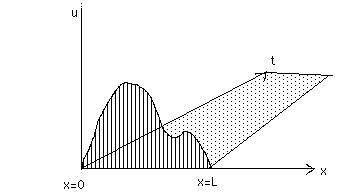We could also have a source term. Physically, this could be thought of as a heater (or refrigerator) adding or removing heat at some rate along the strip. Such an equation could be written as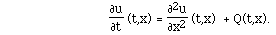Boundary and initial conditions would be as before. In order to rewrite this equation in the context of this course, we should conceive of the equation as L[u] = f , with appropriate boundary conditions. The operator L is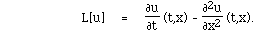This is a parabolic operator according to the definition given above; in fact, the matrix A in (3.1) is given by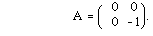(A and Laplaceoperator, or Laplacian: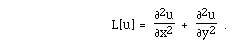Laplace's equation states that

L[u] = 0

and Poisson's equation states that

L[u] = f(x,y)

for some given function f. A physical situation in which it arises is in the problem of finding the shape of a drum under force. Suppose that the bottom of the drum sits on the unit disc in the xy-plane and that the sides of the drum lie above the unit circle. We do not suppose that the sides are at a uniform height, but that the height is specified on the circle.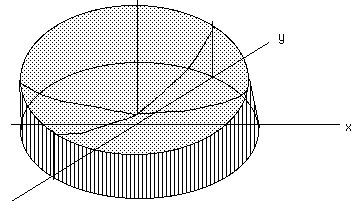That is, we know u(x,y) for {x,y} on the boundary ot the drum. We also suppose that there is a force pulling down, or pushing up, on the drum at each point and that this force is not changing in time. An example of such a force might be the pull of gravity. The question is, what is the shape of the drum? As we shall see, the appropriate equations take the form: Find u if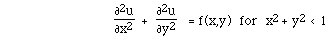with

u(x,y) specified for x2 + y2 = 1.

Laplace's and Poisson's equations also arise in electromagnetism and fluid mechanics as the equations for potential functions. Still another place where you may encounter Laplace's equation is as the equation for a temperature distribution in 2 or 3 dimensions, when thermal equilibrium has been reached. The Laplace operator is elliptic by our definition, for the matrix A in (3.1) is given by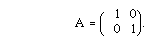Finally, the model for a hyperbolic equation is the one dimensional wave equation. One can think of this equation as describing the motion of a taunt string after an initial perturbation and subject to some outside force. Appropriate boundary conditions are given. To think of this as being a plucked string with the observer watching the up and down motion in time is not a bad perspective, and certainly gives intutive understanding. Here is another perspective, however, which will be more useful in the context of finding the Green function to solve this one dimensional wave equation: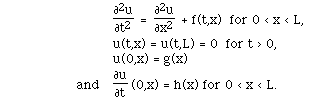As in example (a), the problem is to describe u in the infinite strip within the first quadrant of the xt-plane bounded by the x axis and the lines x = 0 and x = L. Both u and its first derivative in the t direction are known along the x axis. Along the other boundaries, u is zero. What must be the shape of the graph above the infinite strip?

To classify this as a hyperbolic problem, think of the operator L as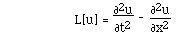and re-write it in the appropriate form for classification. The matrix A in (16.1) is given by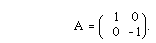There are standard procedures for changing more general partial differential equations to the familiar standard forms, which we shall investigate in the next section.

These very names and ideas suggest a connection with quadratic forms in analytic geometry. We will make this connection a little clearer. Rather than finding geometric understanding of the partial differential equation from this connection we will more likely develop algebraic understanding. Especially, we will see that there are some standard forms. Because of the nearly error free arithmetic that Mapleis able to do, we will offer syntax in Maplethat enables the reader to use this computer algebra system to change second order, linear systems into the standard forms.

If presented with a quadratic equation in x and y, one could likely decide if the equation represented a parabola, hyperbola, or ellipse in the plane. However, if asked to draw a graph of this conic section in the plane, one would start recalling that there are several forms that are easy to draw:

a x2 + b y2 = c2, and the special case x2 + y2 = c2,

a x2 - b y2 = c2, and the special case x2 - y2 = c2,

or
y - a x2 = 0 and x - b y2 = 0.

These quadratic equations represent the familiar conic sections: ellipses, hyperbolas and parabolas, respectively. If a quadratic equation is given that is not in these special forms, then one may recall procedures to transform the equations algebraically into these standard forms. This will be the topic of the next section.

The purpose for doing the classification is that the techniques for solving equations are different in the three classes, if it is possible to solve the equation at all. Even more, there are important resemblance among the solutions of one class; and there are striking differences between the solutions of one class and those of another class. The remainder of these notes will be primarily concerned with finding solutions to hyperbolic, second order, partial differential equations. As we progress, we will see the importance of the equation being a hyperbolic partial differential equation to use the techniques of these notes.

Before comparing the similarity in procedures for changing the partial differential equation to standard form with the preceeding arithmetic, we pause to emphasize the differences in geometry for the types: elliptic, hyperbolic, and parabolic.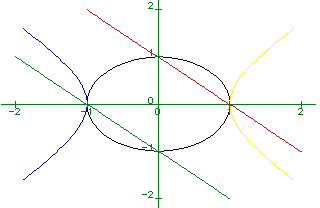Figure 3.1


Here are three equations from analytic geometry:

x2 + y2 = 4 is an ellipse,

x2 - y2 = 4 is a hyperbola,

and x2 + 2 x y + y2 = 4 is a parabola.

Figure 3.1 contains the graphs of all three of these. Their shapes and their geometry, are strikingly different. Even more, naively, one might say that the graph of the third of those above is not the graph of a parabola. Indeed. It does, however, meet the criteria: b2 - 4 a c = 0. One might think of the graph as that of a parabola with vertex at the "point at infinity."

The criterion for classifying second order, partial differential equations is the same: ask what is the character of b2 - 4 a c in the equation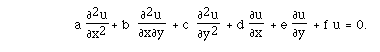We now present solutions for three equations that have the same start -- the same initial conditions. However the equations are of the three types. There is no reason you should know how to solve the three equations yet. There is no reason you should even understand that solving the hyperbolic equation by the method of characteristics is appropriate. But, you should be able to check the solutions -- to see that they solve the specified equations. Each equation has initial conditions

u(0,y) = sin(y) and ux(0,y) = 0.

The equations are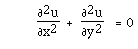Laplace's equation - elliptic;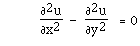a form of the wave equation - hyperbolic; and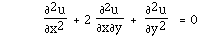is a parabolic partial differential equation,

These have solutions cosh(x) sin(y), cos(x) sin(y), and cos(x-y) x/2 + sin(y-x) respectively. Figure 3.2 has the graphs of these three solutions in order.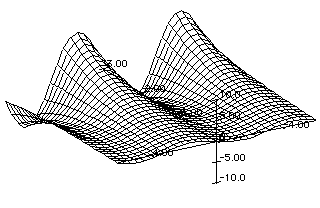Figure 3.2 a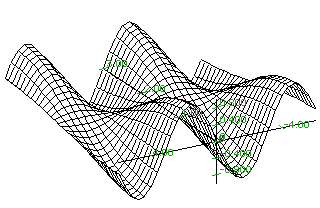Figure 3.2 b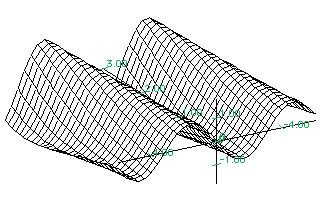Figure 3.2 c


Standard Forms

In this section, we review the procedures to get second-order equations into standard forms, and compare these with the techniques to get second-order partial differential equations with constant coefficients into standard form.

Performing these algebraic procedures corresponds to the geometric notions of translations and rotations.

For example, the equation

x2 - 3y2 - 8x + 30y = 50

represents a hyperbola. To draw the graph of the hyperbola, one algebraically factors the equation or, geometrically, translates the axes:

(x - 4)2 - 3(y - 5)2 = 1.

Now, the response that this is a hyperbola with center {4,5} is expected. More detailed information about the direction of the major and minor axes could be made, but these are not notions that we will wish to carry over to the process of getting second order partial differential equations into standard forms.

There is another idea more appropriate. Rather than keeping the hyperbola in the Euclidean plane where it now has the equation

x2 - 3y2 = 1

in the translated form, think of this hyperbola in the Cartesian plane, and do not insist that the x axis and the y axis have the same scale. In this particular case, keep the x axis the same size and expand the y axis so that every unit is the old unit multiplying by R(3). Algebraically, one commonly writes that there are new coordinates {x',y'} related to the old coordinates by

x = x' , R(3) y = y'.

The algebraic effect is that the equation is transformed into an equation in {x',y'} coordinates:

x' 2 - y' 2 = 1.

Pay attention to the fact that it is a mistake to carry over too much of the geometric language for the form. For example if the original quadratic equation had been

x2 + 3y2 - 8x + 30y = 50

and we had translated axes to produce

x2 + 3y2 = 50,

and then rescaled the axes to get

x2 + y2 = 50

we have not changed an ellipse into a circle for a circle is a geometric object whose very definition involves the notion of distance. The process of changing the scale on the x axis and the y axis certainly destroys the entire notion of distance being the same in all directions.

Rather, the rescaling is an idea that is algebraically simplifying.

Before we pursue the idea of rescaling and translating in second order partial differential equations in order to come up with standard forms, we need to recall that there is also the troublesome need to rotate the axis in order to get some quadratic forms into the standard one. For example, if the equation is

xy = 2,

we quickly recognize this as a quadratic equation. Even more, we could draw the graph. If pushed, we would identify the resulting geometric figure as a hyperbola. We ask for more here since these geometric ideas are more readily transformed into ideas about partial differential equations if they are converted into algebraic ideas. The question, then, is how do we achieve the algebraic representation of the hyperbola in standard form?

One recalls from analytic geometry, or recognizes from simply looking at the picture of the graph of the equation, that this hyperbola has been rotated out of standard form. To see it in standard form, we must rotate the axes. One forgets the details of how this rotation is performed, but should know a reference to find the scheme. From here on, the following may serve as your reference!

Here is the rotation needed to remove the xy term in the equation

a x2 + b xy + c y2 + d x + e y + f = 0.

                                                                       (16.3)


The new coordinates {x', y'} are given by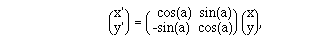(16.4)


where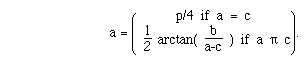(16.5)


And equivalent way to write (11.2) is to multiply both sides by the inverse of the matrix: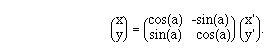(16.6)


Thus, substitute x = x' cos(a) - y' sin(a) and y = x' sin(a) + y' cos(a) into the equation (11.1), where a is as indicated above and the cross term, bxy, will disappear.

Given a general quadratic in two variables, there are three things that need to be done to get it into standard form: get rid of the xy terms, factor all the x terms and the y terms separately, and rescale the axes so that the coefficients of the x2 term and the y2 terms are the same. Geometrically this corresponds, as we have recalled, to a rotation, translation, and expansion, respectively. From the geometric point of view, it does not matter which is done first: the rotation and then the translation, or vice versa. Algebraically, it is better to remove the xy terms first, for then the factoring is easier.

Finally, it should also be remembered that the sign of b2 - 4ac can be used to predict whether the curve is a hyperbola, parabola, or ellipse.

What follows is a Maple program for removing the cross term. Using the program assures fast accurate computation for otherwise tedious calculations to determine the rotation for equations of the form

a x2 + b xy + c y2 +d x +ey + f = 0.

The coefficients are read in first.

* a:=?: b:=?:c:=?:d:=?:e:=?:f:=?:


The angle is determined and the rotation is performed.

*if a=c then alpha := Pi/4
else alpha:=arctan(b/(a-c))/2  fi;
* si:=sin(alpha);
* co:=cos(alpha);
* x:=co*u-si*v; y:=si*u+co*v;
* q:=a*x^2+b*x*y+c*y^2+d*x+e*y+f;
* simplify(");
* evalf(");


The purpose of the previous paragraphs recalling how to change algebraic equations representing two dimensional conic sections into standard form was to suggest that the same ideas carry over almost unchanged for the second degree partial differential equations. The techniques will change these equations into the standard forms for elliptic, hyperbolic, or parabolic partial differential equations.

Here are the forms we want:

Elliptic Equations: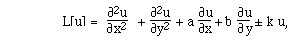Hyperbolic Equations: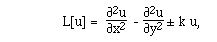Parabolic Equation: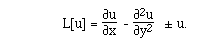The choice for which form to use is determined by the techniques used to solve the equations.

We turn now to the techniques of arriving at standard forms for partial differential equations.

If one has a second order partial differential equation with constant coefficients that is not in the standard form, there is a method to change it into this form. The techniques are similar to those used in the analytic geometry. Having the standard form, one might then solve the equation. Finally, the solution should be transformed back into the original coordinate system.

We will illustrate the procedure for transformation of a second order equation into standard form. Consider the equation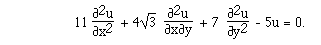In the original equation, if we think of the equation as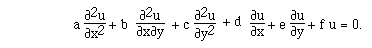Then, a = 11, b = 4 R(3), c = 7, so that b2 - 4 a c = -260 and we identify this as an elliptic equation.

We would like to transform the equation into the form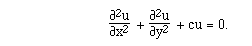Introduce new coordinates (x,h) by rotation of axes so that in the transformed equation the mixed second partial derivative does not appear. Let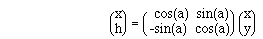or,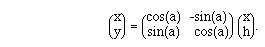Using the chain rule,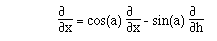and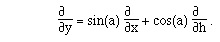It follows that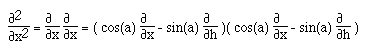so that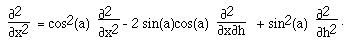In a similar manner,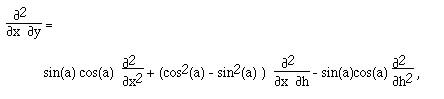and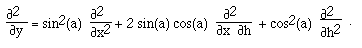The original equation described u as a function of x and y. We now define v as a function of x and h by v(x,h) = u(x,y). The variables x and h are related to x and y as described by the rotation above:

v(x,h) = u(x(x,h), y(x,h))

= u(cos(a) x - sin(a) h, sin(a) x + cos(a) h)

Of course, we have not specified a yet. This comes next.

The equation satisfied by v is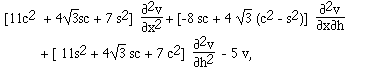where we have used the abbreviations s = sin(a) and c = cos(a). The coefficient of the mixed partials will vanish if a is chosen so that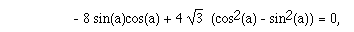that is,

tan(2a) = 31/2/2

This means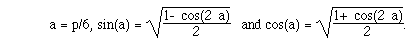After substitution of these values, the equation satisfied by v becomes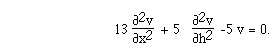This special example, together with the foregoing discussions of analytic geometry makes the following statement believable: Every second order partial differential equation with constant coefficients can be transformed into one in which mixed partials are absent. The angle of rotation is, again, given by (3.3).

It is left as an exercise to see that in the general case, b2 - 4 a c is left unchanged by this rotation. Surely, Maple is to be used to do this calculation. (See Exercise 8.)

We are now ready for the second step: to remove the first order term. For economy of notation, let us assume that the given equation is already in the form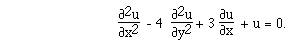Define v by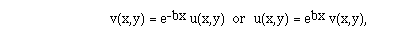where b will be chosen so that the transformed equation will have the first order derivative removed. Differentiating u and substituting into the equation we get that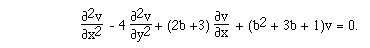If we choose b = - 3/2, we have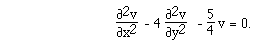Notice that this transformation to achieve an equation lacking the first derivative with respect to x is generally possible when the coefficient on the second derivative with respect to x is not zero, and is otherwise impossible. The same statements hold for derivatives with respect to y.

The final step is rescaling. We choose variables \xi and \eta by

\xi = \mu x and \eta = \nu y, where m and n are chosen so that in the transformed equation the coefficients of v \xi \xi , v \eta \eta , and v are equal in absolute value. We have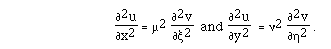Our equation becomesThe condition that

\mu2 = 4 \nu2 = 5/2

will be satisfied if \mu = (5/2)1/2 and \nu = (5/8)1/2 . Then, we obtain the standard form

v \xi \xi - v \eta \eta - v = 0.

Generalized Rotations to Remove Cross Terms

The removal of cross terms as typically presented is complicated by the necessity of finding the angle a of rotation and of working with the ensuing trigonometric functions. These ideas seem inappropriate for higher dimensions.

An alternative approach is to address the problem from the perspective of linear algebra, where the ideas even generalize for large dimensions. We will need the linear algebra package to make this work.

> with(linalg);

We choose the constants as the coefficients for the partial differential equations

a uxx + b xy + cyy + d ux + e uy + f = 0.

> a:=2: b:=3: c:=-2: d:=1: e:=1: f:=3/25:

Make a symmetric matrix with a, b/2, and c as the entries.

> A:=matrix([[a,b/2],[b/2,c]]):

It is a theorem in linear algebra that symmetric matrices have a diagonal Jordan form.

> jordan(A,P);

We want K to be a matrix of eigenvectors.

> K:=inverse(P);

In order to make this process work, we ask that the eigenvectors -- which form the columns of K -- should have norm 1. This will cause the eigenvectors to be orthonormal.

> N1:=norm([K[1,1],K[2,1]],2): N2:=norm([K[1,2],K[2,2]],2):

> L:=matrix([[K[1,1]/N1,K[1,2]/N2],[K[2,1]/N1,K[2,2]/N2]]);

It will now be the case that (transpose(L) * A * L) is the Jordan form.

> evalm(transpose(L) &* A &* L);

To remove the cross-product term, we now perform a change of variables defining s and t.

> s:=(x,y)->evalm(transpose(L) &* vector([x,y])):

t:=(x,y)->evalm(transpose(L) &* vector([x,y])):

If we define v as indicated below, then it will satisfy a PDE with the cross-term missing and with u satisfying the original partial differential equation.

> u:=(x,y)->v(s(x,y),t(x,y)):

> a*diff(u(x,y),x,x)+b*diff(u(x,y),x,y)+c*diff(u(x,y),y,y)+

d*diff(u(x,y),x) + e*diff(u(x,y),y) + u(x,y):

> simplify(");

> collect(",D[1,2](v)(s(x,y),t(x,y)));

> pde:=collect(

collect(

collect(

collect(",D(v)(s(x,y),t(x,y)))

,D(v)(s(x,y),t(x,y)))

,D[2,2](v)(s(x,y),t(x,y)))

,D[1,1](v)(s(x,y),t(x,y)));

Thus, we solve this simpler system and create u that satisfies the original equation.

In the chapters that came before, an understanding of the adjoints of linear functions was critical in determining when certain linear equations would have a solution....and even in computing the solutions for some cases. It is, then, no surprise that we shall be interested in the computation of adjoints in this setting, too.

In the general inner product space, the adjoint of the linear operator L is defined in terms of the dot product:

< L(u) , v > = < u , L*(v) >.

For ordinary differential equations boundary value problems, the dot product came with the problem in a sense: it was an integral over an appropriate interval on which the functions were defined. For partial differential equations with boundary conditions, the dot product will be over the region of interest:

< f , g > = òòD f(x,y) g(x,y) dx dy.

For ordinary differential equations, integration-by-parts played a key role in deciding the appropriate boundary conditions to impose so that the formal adjoint would be the real adjoint. Now, Green's identities provide the appropriate calculus.

In fact, Green's second identity can be used to compute adjoints of the Laplacian. We will see that the divergence theorem is useful for the more general second-order, differential operators.

EXAMPLE: Consider the problem

--2u = f,

u(x,0) = u(x,b) = 0

[[partialdiff]]u/[[partialdiff]]x(0,y) = [[partialdiff]]u/[[partialdiff]]x(a,y) = 0

on an interval [0,a] x [0,b] in the plane. This problem invites consideration of the operator L defined on a manifold as given below:

L(u) = --2u

and M = {u: u(x,0) = u(x,b) = 0, [[partialdiff]]u/[[partialdiff]]y(0,y) = [[partialdiff]]u/[[partialdiff]]y(a,y) = 0}.

The second identity presents a natural setting in which the operator L is self-adjoint in the sense that L = L*. Let u and v be in M:

òòD [v L(u) - L(v) u] dA = ò[[partialdiff]]D [v [[partialdiff]]u/[[partialdiff]][[eta]] - [[partialdiff]]v/[[partialdiff]][[eta]] u] ds = 0.

This last equality follows because, on the boundary, all of u, [[partialdiff]]u/[[partialdiff]][[eta]], v, and [[partialdiff]]v/[[partialdiff]][[eta]] = 0. [[florin]]

In order to discuss adjoints of more general second order partial differential equations, let A, B, C, and c be scalar valued functions. Let b be a vector valued function. Let L(u) be given by

L(u) = A[[partialdiff]]2u/[[partialdiff]]x2 + 2B[[partialdiff]]2u/[[partialdiff]]x[[partialdiff]]y + C[[partialdiff]]2u/[[partialdiff]]y2 + < b , --u > + cu.

DEFINITION: The FORMAL ADJOINT is given by

L*(v) = [[partialdiff]]2(Av)/[[partialdiff]]x2 + 2[[partialdiff]]2(Bv)/[[partialdiff]]x[[partialdiff]]y + [[partialdiff]]2(Cv)/[[partialdiff]]y2 - --*(bv) + cv.

Take A, B, and C to be constant. What would it mean to say that L is formally self-adjoint? That L = L* (formally)? Then <b, --u> must be - --*bu = < -b , --u > - u --*b. Thus, 2 < b , --u > = -u (--*b) for all u. Since this must hold for all u, it must hold in the special case that u [[equivalence]] 1, which implies that --*b = 0. Taking u(x,y) to be x, or to be y gets that each of b1 and b2 = 0. Hence, if L is formally self adjoint, then b = 0.

EXAMPLES:

1. Let L[u] = 3 F([[partialdiff]]2u,[[partialdiff]]x2) + 5 F([[partialdiff]]2u,[[partialdiff]]y2) . The formal adjoint of L is L. Note that

L[u] v - u L[v] = F([[partialdiff]] ,[[partialdiff]]x) ( 3[F([[partialdiff]]u,[[partialdiff]]x) v - u F([[partialdiff]]v,[[partialdiff]]x) ] ) + F([[partialdiff]] ,[[partialdiff]]y) ( 5[F([[partialdiff]]u,[[partialdiff]]y) v - u F([[partialdiff]]v,[[partialdiff]]x) ] )

= --.( 3[F([[partialdiff]]u,[[partialdiff]]x) v - u F([[partialdiff]]v,[[partialdiff]]x) ] , 5[F([[partialdiff]]u,[[partialdiff]]y) v - u F([[partialdiff]]v,[[partialdiff]]x) ] )

2. Let L[u] = 3 F([[partialdiff]]2u,[[partialdiff]]x2) + 5 F([[partialdiff]]2u,[[partialdiff]]y2) + 7 F([[partialdiff]]u,[[partialdiff]]x) + 11 F([[partialdiff]]u,[[partialdiff]]y) + 13 u. The formal adjoint of L is

L*[v] = 3 F([[partialdiff]]2v,[[partialdiff]]x2) + 5 F([[partialdiff]]2v,[[partialdiff]]y2) - 7 F([[partialdiff]]v,[[partialdiff]]x) - 11 F([[partialdiff]]v,[[partialdiff]]y) + 13 v. Note that

L[u] v - u L*[v] = F([[partialdiff]] ,[[partialdiff]]x) ( 3[F([[partialdiff]]u,[[partialdiff]]x) v - u F([[partialdiff]]v,[[partialdiff]]x) ] + 7 uv) + F([[partialdiff]] ,[[partialdiff]]y) ( 5[F([[partialdiff]]u,[[partialdiff]]y) v - u F([[partialdiff]]v,[[partialdiff]]x) ] + 11 uv)

= --.( 3[F([[partialdiff]]u,[[partialdiff]]x) v - u F([[partialdiff]]v,[[partialdiff]]x) ] +7 uv, 5[F([[partialdiff]]u,[[partialdiff]]y) v - u F([[partialdiff]]v,[[partialdiff]]x) ] + 11 uv).

3. Let L[u] = ex F([[partialdiff]]2u,[[partialdiff]]x2) + 5 F([[partialdiff]]u,[[partialdiff]]y) + 3u. The formal adjoint of L is L* given by

L*[v] = ex F([[partialdiff]]2v,[[partialdiff]]x2) + 2ex F([[partialdiff]]v,[[partialdiff]]x) -5F([[partialdiff]]v,[[partialdiff]]y) + (ex+3) u. Note that

L[u] v - u L*[v] = F([[partialdiff]] ,[[partialdiff]]x) (ex v F([[partialdiff]]u,[[partialdiff]]x) - u F([[partialdiff]] exv,[[partialdiff]]x) ) + F([[partialdiff]] ,[[partialdiff]]y) (5uv)

= --.( ex v F([[partialdiff]]u,[[partialdiff]]x) - u F([[partialdiff]] exv,[[partialdiff]]x) , 5uv).

THE CONSTRUCTION OF M*

We now come to the important part of the construction of the real adjoint: how to construct the appropriate adjoint boundary conditions. We are given L and M; we have discussed how to construct L*. We now construct M*. To see what is M* in the general case, the divergence theorem is recalled:

òòD --*F dx dy = ò[[partialdiff]]D < F, [[eta]] > ds.

The hope, then, is to write v L(u) - L*(v) u as --*F for some suitable chosen F.

Theorem. If L is a second order differential operator and L* is the formal adjoint, then there is F such that vL(u) - L*(v)u = --.F.

Here's how to see that. Note that

(v A[[partialdiff]]2u/[[partialdiff]]x2 + v C[[partialdiff]]2u/[[partialdiff]]y2) - (u [[partialdiff]]2(Av)/[[partialdiff]]x2 + u [[partialdiff]]2(Cv)/[[partialdiff]]y2)

= [[partialdiff]]/[[partialdiff]]x(vA[[partialdiff]]u/[[partialdiff]]x - u[[partialdiff]](Av)/[[partialdiff]]x) + [[partialdiff]]/[[partialdiff]]y (vC[[partialdiff]]u/[[partialdiff]]y - u[[partialdiff]](Cv)/[[partialdiff]]y)

= --*{ v(A[[partialdiff]]u/[[partialdiff]]x, C[[partialdiff]]u/[[partialdiff]]y) - u([[partialdiff]]Av/[[partialdiff]]x, [[partialdiff]]Cv/[[partialdiff]]y)}.

Also, v < b , --u > + u --*(bv)

= v b1 [[partialdiff]]u/[[partialdiff]]x + b2 [[partialdiff]]u/[[partialdiff]]y v + u [[partialdiff]](b1v)/[[partialdiff]]x + u [[partialdiff]](b2v)/[[partialdiff]]y

= --*(vbu).

COROLLARY: òòD [vL(u) - uL*(v)]

= òòD --*{v(A[[partialdiff]]u/[[partialdiff]]x,C[[partialdiff]]u/[[partialdiff]]y) - u([[partialdiff]](Av)/[[partialdiff]]x,[[partialdiff]](Cv)/[[partialdiff]]y) + vbu)

=ò[[partialdiff]]D [v{A[[partialdiff]]u/[[partialdiff]]x,C[[partialdiff]]u/[[partialdiff]]y}-u{[[patialdiff]](Av)[[partialdiff]]x,[[partialdiff]](Cv)/[[partialdiff]]y} +vbu}]*[[eta]] ds.

EXAMPLES.

1(cont). Let L[u] be as in example 1 above for {x,y} in the rectangle D = [0,1]x[0,1] and M = {u: u=0 on [[partialdiff]]D}. Then, according to Example 1, L = L* and

òòD [vL(u) - uL*(v)] dA=

ò[[partialdiff]]D <{3 [ F([[partialdiff]]u,[[partialdiff]]x) v - u F([[partialdiff]]v,[[partialdiff]]x) ], 5 [ F([[partialdiff]]u,[[partialdiff]]y) v - u F([[partialdiff]]v,[[partialdiff]]y)] } , [[eta]] > ds.

Recalling that the unit normal to the faces of the rectangle D will be {0.-1}, {1,0}, {0,1}, or {-1,0} and that u = 0 on [[partialdiff]]D, we have that

òòD [vL(u) - uL*(v)] dA=

= - I(0,1, ) 5F([[partialdiff]]u,[[partialdiff]]y) v dx + 3 I(0,1, )F([[partialdiff]]u,[[partialdiff]]x) v dy

+ 5 I(1,0, ) F([[partialdiff]]u,[[partialdiff]]y) v |dx| - 3 I(1,0, ) F([[partialdiff]]u,[[partialdiff]]y) v |dy|.

In order for this integral to be zero for all u in M, it must be that v = 0 on [[partialdiff]]D. And M = M*. Hence, {L, M} is (really) self adjoint.

2.(cont) Let L[u] be as in Example 2 above and M = {u: u(x,0) = u(0,y) = 0, and F([[partialdiff]]u,[[partialdiff]]x)(1,y) = F([[partialdiff]]u,[[partialdiff]]y)(x,1) = 0, 0 < x < 1, 0 < y < 1}. Using the results from above,

òòD [L(u)v - uL*(v)] dA=

-I(0,1, ) 5 F([[partialdiff]]u,[[partialdiff]]y) v dx + I(0,1, ) [-3 u F([[partialdiff]]v,[[partialdiff]]x) + 7uv] dy

+ I(1,0, ) [5u F([[partialdiff]]v,[[partialdiff]]x) + 11 uv] |dx| - I(1,0, )[3 F([[partialdiff]]u,[[partialdiff]]x) v ] |dy|.

It follows that M* = {v: v(x,0) = 0, v(1,y) = F(3,7) F([[partialdiff]]v,[[partialdiff]]x) (1,y), v(x,1) =

F(5,11) F([[partialdiff]]v,[[partialdiff]]x) (x,1), and v(0,y) = 0}.

3(cont). Let L[u] be as in Example 3 above for [x,y} in the first qaudrant. Let M = {u: u = 0 on [[partialdiff]]D}. Then

òòD [vL(u) - uL*(v)] dA=

=ò[[partialdiff]]D <{ex v F([[partialdiff]]u,[[partialdiff]]x) - u F([[partialdiff]] exv ,[[partialdiff]]x) , 5uv }, [[eta]] > ds = - I(0,*, )v F([[partialdiff]]u,[[partialdiff]]x) dy.

Thus, M* = {v: v(0,y) = 0 for y > 0}.

Exercises XVI

XVI.1.    Classify each of the following as hyperbolic, parabolic, or elliptic.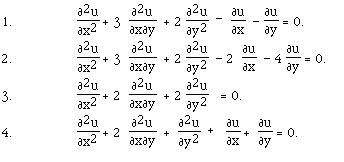XVI.5.    Transform the following equations into standard form: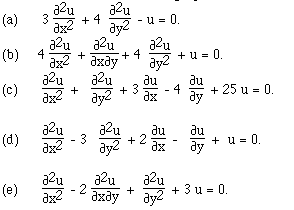XVI.6.    Show that the equation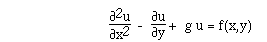where g is any constant, can be transformed into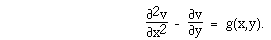XVI.7.    Show that by rotation of the axis by 45 degrees the equations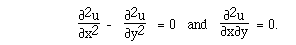can be transformed into one another. Find the general solution for both equations.

XVI.8.    Show that upon making a rotation to remove the cross terms, then the discriminant b2 - 4ac is unchanged.

XVI.9.    Suppose that L(u) = [[partialdiff]]2u/[[partialdiff]]x2 - [[partialdiff]]2u/[[partialdiff]]t2 restricted to M = {u: u(0,t) =u(a,t) = 0 and u(x,0) = [[partialdiff]]u/[[partialdiff]]t(x,0) = 0}. Classify L as parabolic, hyperbolic, or elliptic. Find L* . Find F such that v L[u] - L*[v} u = --F. What is M*?

XVI.10.    Suppose that L[u] = [[partialdiff]]2u/[[partialdiff]]x2 + [[partialdiff]]2u/[[partialdiff]]y2 - [[partialdiff]]2u/[[partialdiff]]z2 restricted to

M = {u: u(0,y,z) = 0, u(1,y,z) = 0, u(x,y,0) = u(x,y,1), [[partialdiff]]u/[[partialdiff]]z (x,y,0) = [[partialdiff]]u/[[partialdiff]]z(x,y,1),

[[partialdiff]]u/[[partialdiff]]y(x,0,z) = 3 u(x,0,z), [[partialdiff]]u/[[partialdiff]]y(x,1,z) = 5 u(x,1,z)}

Classify L as parabolic, hyperbolic, or elliptic. Give L*. Find F such that v L[u} - L*[v] u = --.F. What is M*?

Onward to Chapter XIX Back to Chapter XVII